• +91 9971497814
• info@interviewmaterial.com

# RD Chapter 1- Real Numbers Ex-MCQS Interview Questions Answers

### Related Subjects

Question 1 :
The exponent of 2 in the prime factorisation of 144, is
(a) 4
(b) 5
(c) 6
(d) 3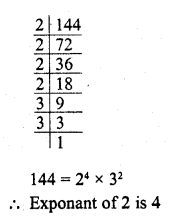Question 2 :
The LCM of two numbersls 1200. Which of the following cannot be their HCF ?
(a) 600
(b) 500
(c) 400
(d) 200

(b) LCM of two number = 1200
Their HCF of these two numbers will be the factor of 1200
500 cannot be its HCF

Question 3 :
If n = 23 x 34 x 44 x 7, then the number of consecutive zeroes in n, where n is a natural number, is
(a) 2
(b) 3
(c) 4
(d) 7

(c) Because it has four factors n = 23 x34 x 44 x 7
It has 4 zeroes

Question 4 :
The sum of the exponents of the prime factors in the prime factorisation of 196, is
(a) 1
(b) 2
(c) 4
(d) 6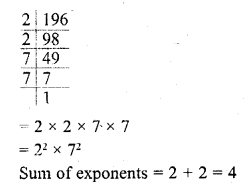Question 5 :
The number of decimal places after which the decimal expansion of the rational number 23/22×5 will terminate, is
(a) 1
(b) 2
(c) 3
(d) 4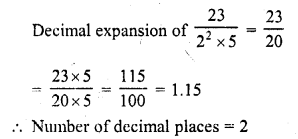Question 6 :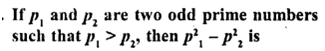(a) an even number
(b) an odd number
(c) an odd prime number
(d) a prime number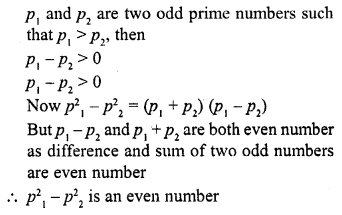Question 7 : If two positive integers a and b are expressible inthe form a = pq2 and b = p2q ; p, q being primenumbers, then LCM (a, b) is
(a) pq
(b) p3q3
(c) p3q2
(d) p2q2

(c) a and b are two positive integers anda =pq2 and b = p3q, where p and q are prime numbers,then LCM=p3q2

Question 8 : In Q. No. 7, HCF (a, b) is
(a) pq
(b) p3q3
(c) p3q2
(d) p2q2

(a) a = pq2 and b =p3qwhere a and b are positive integers and p, q are prime numbers, then HCF =pq

Question 9 : If two positive integers tn and n arc expressible inthe form m = pq3 and n = p3q2, where p, qare prime numbers, then HCF (m, n) =
(a) pq
(b) pq2
(c) p3q3
(d) p2q3

(b) m and n are two positive integers andm = pq3 and n = pq2, where p and q are primenumbers, then HCF = pq2

Question 10 :
If the LCM of a and 18 is 36 and the HCF of a and 18 is 2, then a =
(a) 2
(b) 3
(c) 4
(d) 1

(c) LCM of a and 18 = 36
and HCF of a and 18 = 2
Product of LCM and HCF = product of numbers
36 x 2 = a x 18
a = 36×2/18 = 4

Todays Deals### RD Chapter 1- Real Numbers Ex-MCQS Contributorskrishan

Name:
Email:

# Latest News# 9000 interview questions in different categories